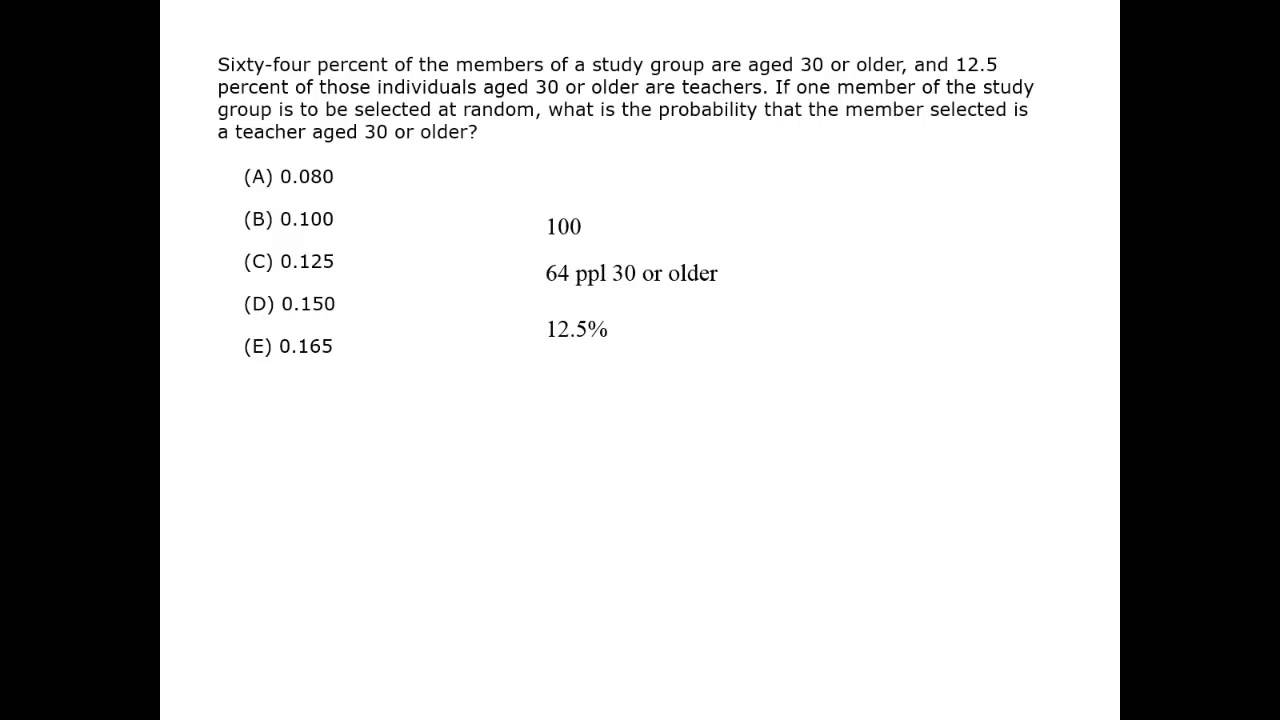The probability of an event is a number between 0 and 1 that describes the likelihood that the event will occur. Events can also be called outcomes or possibilities.

Probability can be given as a fraction (\dfrac{2}{5}), a ratio (2 in 5) or a percent (40%).

Probability

A class has 17 girls and 17 boys. If a student is called at random, what is the probability it will be a girl?

### Solution

There are 34 possible outcomes. The desired outcome is a girl being called.

Bottom: 34 outcomes are possible
Top: 17 possible desired outcomes
Probability: \dfrac{17}{34} = \dfrac{1}{2}

Or, more simply:

probability = \dfrac{desired\, outcome}{all\, possible\, outcomes}

Apply that to the sample of rolling a 5.

Bottom: 6 outcomes are possible
Top: 1 desired outcome
Probability: \dfrac{1}{6}

#### 800score Technique: The Bottom and the Top

If you want to make probability simpler, just think of it as a fraction to define Bottom then Top. The bottom number is the total number of possibilities that could happen, and the top number is the number of possible ways to achieve the desired result.

Video Courtesy of Kaplan GMAT prep.

Probability is often seen as a very difficult part of the GMAT, but by logically applying one simple fraction you can make probability much easier. Start with probability in its most basic form.

You have a fair six-sided die. If you roll it once, what is the probability you roll a 5?

There are 6 sides to the die, and each is equally likely to come up. So there are 6 possible outcomes, and rolling a 5 is the one that you want. Therefore, there is a 1 in 6 probability that the 5 will come up. (Note: die is the singular of dice.)

A fruit basket has 10 apples, 8 oranges and 6 pears. What is the probability of randomly picking an orange?

### Solution

There are 24 possible outcomes. An orange is wanted.

Bottom: 24 outcomes are possible
Top: 8 of the fruits are oranges (desired outcome)
Probability: \dfrac{8}{24} = \dfrac{1}{3}

A bag contains 3 white marbles, 2 blue marbles and 5 red marbles. What is the percent probability of drawing a white marble from the bag?

### Solution

There are a total of 10 marbles in the bag.

Bottom: 10 outcomes are possible
Top: 3 marbles are white
Probability: \dfrac{3}{10} = 30%

Example – Selecting marbles from a bag

#### Simple Probability

Best viewed in landscape mode

2 questions with video explanations

100 seconds per questionBefore attempting these problems, be sure to review this section on data sufficiency questions.